x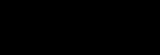METEOREncyclopedia
METEOR is a metric
Metric (mathematics)
In mathematics, a metric or distance function is a function which defines a distance between elements of a set. A set with a metric is called a metric space. A metric induces a topology on a set but not all topologies can be generated by a metric...

for the evaluation of machine translation
Machine translation
Machine translation, sometimes referred to by the abbreviation MT is a sub-field of computational linguistics that investigates the use of computer software to translate text or speech from one natural language to another.On a basic...

output. The metric is based on the harmonic mean
Harmonic mean
In mathematics, the harmonic mean is one of several kinds of average. Typically, it is appropriate for situations when the average of rates is desired....

of unigram precision and recall
Precision and recall
In pattern recognition and information retrieval, precision is the fraction of retrieved instances that are relevant, while recall is the fraction of relevant instances that are retrieved. Both precision and recall are therefore based on an understanding and measure of relevance...

, with recall weighted higher than precision. It also has several features that are not found in other metrics, such as stemming
Stemming
In linguistic morphology and information retrieval, stemming is the process for reducing inflected words to their stem, base or root form—generally a written word form. The stem need not be identical to the morphological root of the word; it is usually sufficient that related words map to the same...

and synonymy matching, along with the standard exact word matching. The metric was designed to fix some of the problems found in the more popular BLEU
Bleu
bleu or BLEU may refer to:* the French word for blue* Three Colors: Blue, a 1993 movie* Bilingual Evaluation Understudy, a machine translation evaluation metric* Belgium–Luxembourg Economic Union...

metric, and also produce good correlation with human judgement at the sentence or segment level This differs from the BLEU metric in that BLEU seeks correlation at the corpus level.Results have been presented which give correlation
Pearson product-moment correlation coefficient
In statistics, the Pearson product-moment correlation coefficient is a measure of the correlation between two variables X and Y, giving a value between +1 and −1 inclusive...

of up to 0.964 with human judgement at the corpus level, compared to BLEU
Bleu
bleu or BLEU may refer to:* the French word for blue* Three Colors: Blue, a 1993 movie* Bilingual Evaluation Understudy, a machine translation evaluation metric* Belgium–Luxembourg Economic Union...

's achievement of 0.817 on the same data set. At the sentence level, the maximum correlation with human judgement achieved was 0.403.## Algorithm

As with BLEU
Bleu
bleu or BLEU may refer to:* the French word for blue* Three Colors: Blue, a 1993 movie* Bilingual Evaluation Understudy, a machine translation evaluation metric* Belgium–Luxembourg Economic Union...

, the basic unit of evaluation is the sentence, the algorithm first creates an alignment (see illustrations) between two sentence
Sentence (linguistics)
In the field of linguistics, a sentence is an expression in natural language, and often defined to indicate a grammatical unit consisting of one or more words that generally bear minimal syntactic relation to the words that precede or follow it...

s, the candidate translation string, and the reference translation string. The alignment is a set of mappings between unigrams. A mapping can be thought of as a line between a unigram in one string, and a unigram in another string. The constraints are as follows; every unigram in the candidate translation must map to zero or one unigram in the reference. Mappings are selected to produce an alignment as defined above. If there are two alignments with the same number of mappings, the alignment is chosen with the fewest crosses, that is, with fewer intersections
Intersection (set theory)
In mathematics, the intersection of two sets A and B is the set that contains all elements of A that also belong to B , but no other elements....

of two mappings. From the two alignments shown, alignment (a) would be selected at this point. Stages are run consecutively and each stage only adds to the alignment those unigrams which have not been matched in previous stages. Once the final alignment is computed, the score is computed as follows: Unigram precision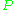is calculated as:
will be mapped by each module
|-
! Module || Candidate || Reference || Match
|-
| Exact || good || good || Yes
|-
| Stemmer || goods || good || Yes
|-
| Synonymy || well || good || Yes
|-
|}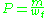Whereis the number of unigrams in the candidate translation that are also found in the reference translation, and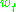is the number of unigrams in the candidate translation. Unigram recall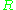is computed as: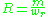Whereis as above, and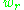is the number of unigrams in the reference translation. Precision and recall are combined using the harmonic mean
Harmonic mean
In mathematics, the harmonic mean is one of several kinds of average. Typically, it is appropriate for situations when the average of rates is desired....

in the following fashion, with recall weighted 9 times more than precision: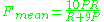The measures that have been introduced so far only account for congruity with respect to single words but not with respect to larger segments that appear in both the reference and the candidate sentence. In order to take these into account, longer n-gram matches are used to compute a penalty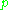for the alignment. The more mappings there are that are not adjacent in the reference and the candidate sentence, the higher the penalty will be.

In order to compute this penalty, unigrams are grouped into the fewest possible chunks, where a chunk is defined as a set of unigrams that are adjacent in the hypothesis and in the reference. The longer the adjacent mappings between the candidate and the reference, the fewer chunks there are. A translation that is identical to the reference will give just one chunk. The penaltyis computed as follows,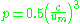Where c is the number of chunks, and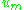is the number of unigrams that have been mapped. The final score for a segment is calculated as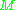below. The penalty has the effect of reducing the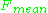by up to 50% if there are no bigram or longer matches.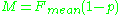To calculate a score over a whole corpus
Text corpus
In linguistics, a corpus or text corpus is a large and structured set of texts...

, or collection of segments, the aggregate values for,andare taken and then combined using the same formula. The algorithm also works for comparing a candidate translation against more than one reference translations. In this case the algorithm compares the candidate against each of the references and selects the highest score.

## Examples

 Reference the cat sat on the mat Hypothesis on the mat sat the cat

```
Score: 0.5000 = Fmean: 1.0000 * (1 - Penalty: 0.5000)
Fmean: 1.0000 = 10 * Precision: 1.0000 * Recall: 1.0000 / Recall: 1.0000 + 9 * Precision: 1.0000
Penalty: 0.5000 = 0.5 * (Fragmentation: 1.0000 ^3)
Fragmentation: 1.0000 = Chunks: 6.0000 / Matches: 6.0000
```

 Reference the cat sat on the mat Hypothesis the cat sat on the mat

```
Score: 0.9977 = Fmean: 1.0000 * (1 - Penalty: 0.0023)
Fmean: 1.0000 = 10 * Precision: 1.0000 * Recall: 1.0000 / Recall: 1.0000 + 9 * Precision: 1.0000
Penalty: 0.0023 = 0.5 * (Fragmentation: 0.1667 ^3)
Fragmentation: 0.1667 = Chunks: 1.0000 / Matches: 6.0000
```

 Reference the cat sat on the mat Hypothesis the cat was sat on the mat

```
Score: 0.9654 = Fmean: 0.9836 * (1 - Penalty: 0.0185)
Fmean: 0.9836 = 10 * Precision: 0.8571 * Recall: 1.0000 / Recall: 1.0000 + 9 * Precision: 0.8571
Penalty: 0.0185 = 0.5 * (Fragmentation: 0.3333 ^3)
Fragmentation: 0.3333 = Chunks: 2.0000 / Matches: 6.0000
```

• BLEU
Bleu
bleu or BLEU may refer to:* the French word for blue* Three Colors: Blue, a 1993 movie* Bilingual Evaluation Understudy, a machine translation evaluation metric* Belgium–Luxembourg Economic Union...

• F-Measure
F1 Score
In statistics, the F1 score is a measure of a test's accuracy. It considers both the precision p and the recall r of the test to compute the score: p is the number of correct results divided by the number of all returned results and r is the number of correct results divided by the number of...

• NIST (metric)
NIST (metric)
NIST is a method for evaluating the quality of text which has been translated using machine translation. Its name comes from the US National Institute of Standards and Technology....

• ROUGE (metric)
ROUGE (metric)
ROUGE, or Recall-Oriented Understudy for Gisting Evaluation, is a set of metrics and a software package used for evaluating automatic summarization and machine translation software in natural language processing...

• Word Error Rate (WER)
Word error rate
Word error rate is a common metric of the performance of a speech recognition or machine translation system.The general difficulty of measuring performance lies in the fact that the recognized word sequence can have a different length from the reference word sequence...

• Noun-Phrase Chunking